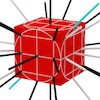# Proof of the Kochen-Specker Theorem

Rachel Thomas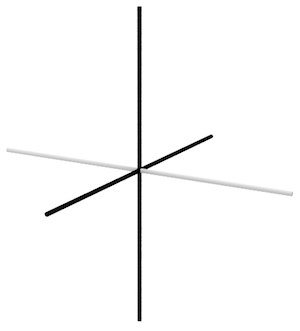The 101 property has an immediate consequence: the squared spin of a particle has the same value for opposite directions. To see this imagine measuring the squared spin of a particle in the three perpendicular directions along the positive x, y, and z axes. Suppose it is 1 in the positive x direction (shown as the black axis pointing out of the page), 0 in the positive y direction (show as the white axis pointing to the right), and 1 in the positive z direction (the black axis pointing upwards), satisfying the 101 property.

Then we can also measure the squared spin in the three perpendicular directions along the positive x and y axes, and the negative z axis (pointing down). Then as the squared spin is 1 in the positive x direction and 0 in the positive y direction, then by the 101 property it must be 1 in the negative z direction. The same argument can be used for any other direction.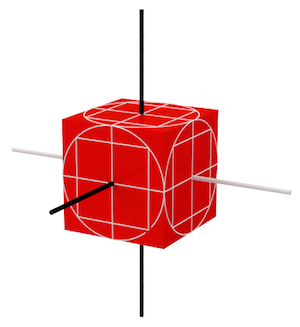Kochen and Specker’s ingenious argument was to consider the squared spin in just a handful of directions. The simplest version of their result (discovered by Asher Peres) drew two lines dividing each face in quarters, then drew the largest possible circle on each face, and inside of each of these circles drew the largest possible square.

They only used the directions from the particle at the centre of the cube to the intersections of these markings on the faces: 33 directions in all. (There are 9 intersections on each of the 6 faces and an additional point on the middle of each of the 12 edges, which creates 66 directions from the centre of the cube in total. But as the squared spin is the same in opposite directions, Peres really only had to consider half of these, the intersections on 3 of the faces.)

The proof takes the form of a proof by contradiction. First we assume that the value of the squared spin for a particle has a pre-existing definite value for every possible direction. We then start with three perpendicular directions. By the 101 property, the squared spin of the particle will be 1 in two of these directions (shown by black knitting needles pushed through the cube) and 0 in the other (shown as a white knitting needle).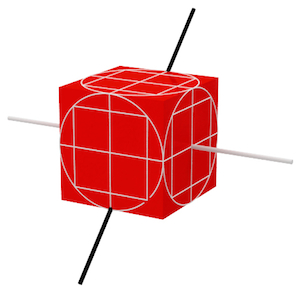Then, again by the 101 property, squared spin of the particle will be 0 in every direction perpendicular to the first white needle. For example, the squared spin will be 1 in the direction from the particle to the middle of the top-rear edge of the cube (shown as a black needle pushed through the cube).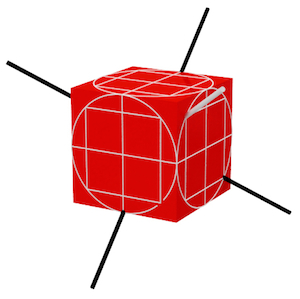This last direction, through middle of the top-rear edge of the cube, is mutually perpendicular with to the needles through the top-left and bottom-right corners of the squares on the right-side face of the cube. So the squared spin in one of these directions is 1 and is 0 in the other. We can colour the top-left needle white and the bottom-right needle black the squared spin in one of these. If it was the other way around, symmetry means you can just roll the cube 180 degrees forward (on to the top face) to give this configuration. (A similar argument shows the top-right needle on the right-side face would also be white.)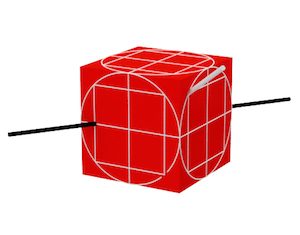Then, by the 101 property, the squared spin will be 1 in any direction perpendicular to the top-left needle through the right-side face. For example, the needle through the middle-left side of the square on the front-side of the cube will be black.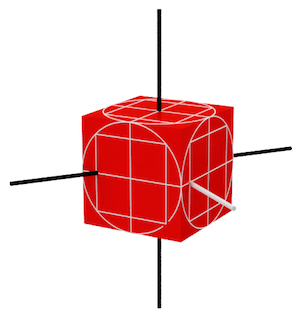Then this black middle-left needle through the front-face of the cube is perpendicular to the previous black needle through the centre of the top face. These needles are mutually perpendicular to that through the middle-left of the right-side face, and this needle must therefore be white. (A similar argument shows the middle-right needle through the right-side face is also white.)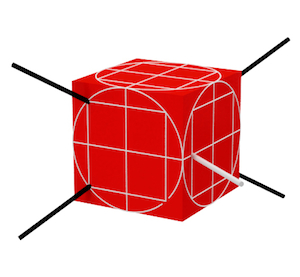Then by the 101 property all needles perpendicular to this white needle must be black – including the top-left and bottom-left needles through the front face of the cube. (Similarly the top-right and bottom-right needles through the front face must also be black, as they are both perpendicular to the white middle-right needle through the right-side face of the cube.)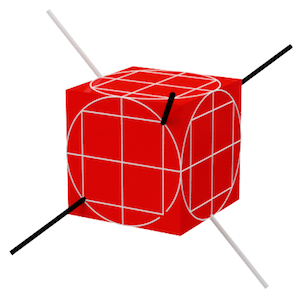Then the bottom-left and top-right needles through the front face of the cube are mutually perpendicular to the needle through the middle of the top-left edge of the cube. Therefore this needle must be white.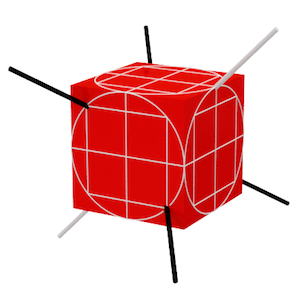Similarly, the top-left and bottom-right needles are mutually perpendicular to the needle through the middle of the top-right edge of the cube. Therefore this needle must also be white.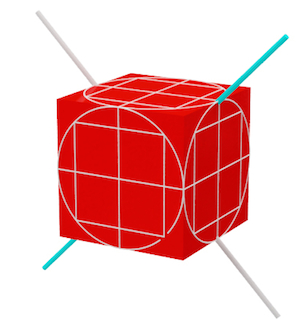The white needle through the middle of the top-left edge of the cube is perpendicular to the needle through the middle of the top-right edge, which implies that this needle must be black. But we have just seen, shown in the picture above, that this needle must be white. And we have reached a contradiction: this needle (shown in blue) must be both black and white.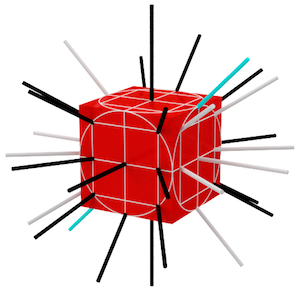If you assume that the squared spin of the particle has a definite value in all of these directions, you end up with a contradiction: the squared spin in one direction ends up being both 0 and 1 (indicated by the blue needle).

“If the answers were chosen ahead of time you could stick a knitting needle through each of these directions,” says Conway. “Thirty-three knitting needles: a black needle if the predetermined answer was 1 and white if the answer was 0. But [Kochen and Specker showed that] it is impossible to label all these directions with 1’s and 0’s so that they all satisfy the 101 rule; you get to a point when you need both a black and white needle.”

Back to the article